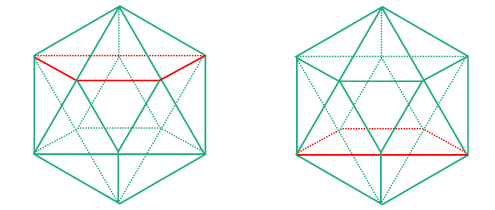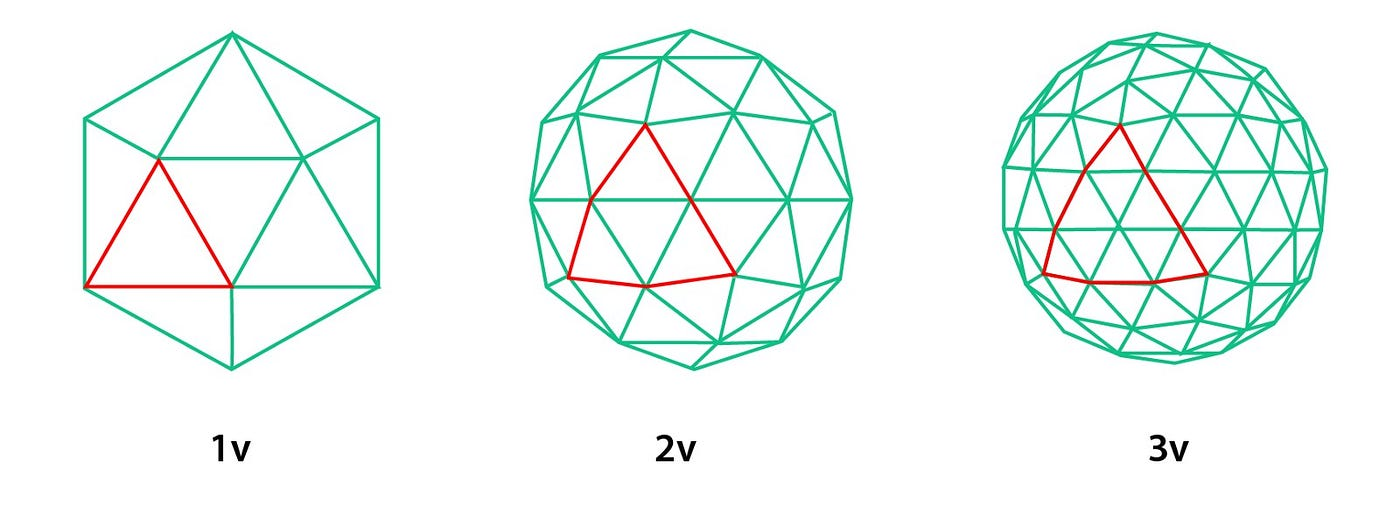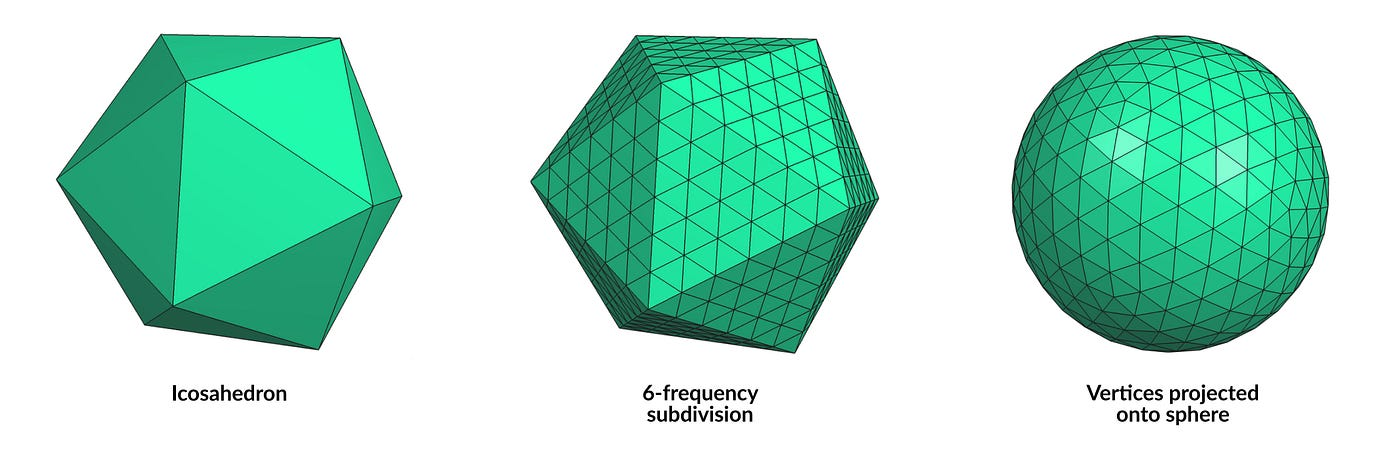# What is a Geodesic Dome?

A geodesic dome is essentially an approximation of a hemisphere by a series of triangles, mathematically described as a number of points located on the surface of a hemisphere. We group these points into triples, connecting the three points with straight lines which form the edges of the triangles and the bars of the dome, giving it it’s characteristic strength. But how do we know where to locate these points on the hemisphere? How do we know if this structure gives us a reasonable approximation of a hemisphere? How do we build a geodesic dome mathematically knowing nothing about it? The answer lies over two millennia ago in Ancient Greece. The Greeks, being at the forefront of mathematics of their time, researched a number of mathematical phenomena, including various geometries and shapes. Some of these shapes they described in their writings were known as Platonic Solids, named after the Greek philosopher Plato.

They were known as Platonic Solids, as Plato theorised that the five Platonic Solids made up the world that we know, with each of the five platonic solids representing one of the classical elements (fire, water, earth etc). One of these solids, called the icosahedron, forms the foundation for our geodesic dome. The icosahedron is solid that can be constructed from triangles, having 12 vertices, 30 edges, and 20 faces. Each of these vertices lies on the surface of the circumscribed sphere (a mathematical sphere that surrounds the dome and helps us in constructing it), which will later become the hemisphere that our dome will be approximating. The first step from our icosahedron to our dome is to cut the shape, getting us from approximating a sphere to approximating a hemisphere. Where to cut the dome depends on personal preference, and as we will encounter soon, the frequency of the geodesic shape descended from the icosahedron. For the simplest frequency ν=1, a regular icosahedron, we can either cut the shape at the bottom of the first set of triangles from the top point or at the bottom of the next set of triangles.Frequency cut sections of both the top section and bottom section of an icosahedron

The first option gives a flatter dome, while the second options give higher but more vertical walls around the dome. Cutting in the exact middle of the icosahedron cuts our triangles into two pieces, leaving edges unconnected and the resulting design weak and inelegant. Here we come upon one of the fundamentals geodesic dome construction, the frequency of the geodesic dome. As each face of the icosahedron is a triangle, we can choose to subdivide the triangle into smaller triangles.Each face of the original icosahedron is subdivided into multiple triangles

The number of triangles follows from our chosen frequency for the dome. For a frequency ν=1 our original triangles in our icosahedron remain as they were, frequency ν=2 splits each original triangle in the icosahedron into 4 smaller triangles, ν=3 splits it into 9 triangles, and so on and so forth.

Left alone, the frequency does nothing to aid our approximation of a hemisphere — the faces are still that of the original icosahedron, just split into more triangles. The key comes when, matching the original points of the icosahedron, we push out every point created by our new triangles onto the circumscribed sphere given to us from our original icosahedron.Pushing the vertices out to the circumscribed sphere for a ν=6 icosahedron

This creates more points that are on the edge of the circumscribed sphere, improving our approximation of the hemisphere in our dome. Knowing this, we can assert that the higher the frequency of our dome, the closer our approximation of a hemisphere. For even frequencies the problem of cutting our new geodesic object into a hemisphere is easy, we can cut along the edge located in the middle of the object, like the equator on Earth, giving a geodesic dome with a flat base. For odd frequencies, we are left with the same choice as the original icosahedron, either a smaller and flatter dome or a larger dome (cutting below the equator of the object) with more vertical walls/faces around the perimeter. Knowing all of this we have created the blueprints for a geodesic dome, as there are many easy to find mathematical representations of an icosahedron in either Cartesian or polar coordinates, and the subsequent subdivisions of the triangles are easy to calculate using rudimentary mathematics.

As an aside, many of the representations of the icosahedron found on the internet give us an edge at the top of our object, which is not useful as we want our dome to have a point at the top, allowing us to have a flat base for ease of construction. While one can take the polar coordinates and compute them as Cartesian coordinates, another way is to rotate all the points found on the sphere using an object in linear algebra called a rotation matrix. Using this method, one finds the two highest points on the vertical axis and finds the horizontal axis that is perpendicular to the edge between the two highest points. Calculating the angle between either one of these vertices and the edge of the circle located on the vertical axis, one simply applies this angle to the appropriate rotational matrix given our perpendicular horizontal axis, resulting in a single point that lies directly on the vertical axis and is the highest along said axis, giving us a point at the crest of the dome, and now a flat base.

--

--

--

## More from OneIsland

We create organic and biomorphic housing structures. We’re reconstructing how we as people live.

## They’re not soft skills – they’re really hard skills## Concept validation: The perfect UX Research midway method## A Design Sprint team is greater than the sum of its parts.## “Finally” vs. “Yet another”## What Can Design Do for You During a Pandemic? Inner World Travel.## Case study: Designing a meal ordering app from figma## Web Design vs. Graphic Design: How are They Different?## Type & Hierarchy — Avenir## The Trap Of L’Hôpital’s Rule## Division Definition, Symbol, Examples## Modular Arithmetic